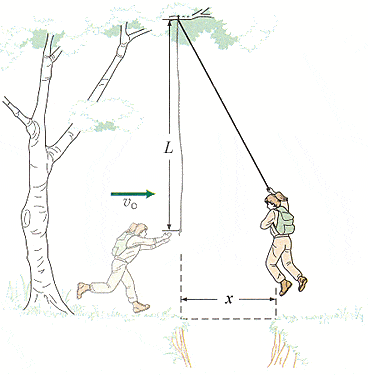# Swinging on a rope problem

Iconic

## Homework Statement

A hiker plans to swing on a rope across a ravine in the mountains, as illustrated in the figure, where L = 4.0 m and x = 1.8 m, and to drop when she is just above the far edge. At what minimum horizontal speed should she be moving when she starts to swing(in m/s)?Ei= Ef
So...
Ke = Pe
So...
1/2mv2=mgh

## The Attempt at a Solution

I have the equation set up correctly but I just don't know how to find h so I can find V

1/2mv2=mgh

The Rope makes a isosceles triangle shape so I thought of L as adjacent, and X as the Opposite so I could solve for theta by doing Θ = arctan(1.8/4.0) = 24.22º. However, upon looking at the answer online- it says to take the arcsin(1.8/4.0) = 26.7º. Why is this?

Last edited: